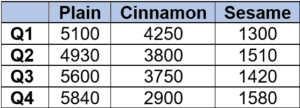# PCAT Quantitative Reasoning – Bagel Stand Profits

The quarterly sales at a bagel stand are shown in the table below. The bagel stand sells plain bagels for \$1.50 each, cinnamon bagels for \$1.80 each, and sesame bagels for \$1.60 each. If it costs the bagel stand \$0.50 to make each bagel, which quarter had the highest profit?1. Q1
2. Q2
3. Q3
4. Q4

##### Explanation

A is correct. To solve, which can simply calculate the profit in each quarter using a calculator. First we must take into account the \$0.50 manufacturing cost, so the profit per bagel is:

Plain: \$1.50 – \$0.50 = \$1.00

Cinnamon = \$1.80 – \$0.50 = \$1.30

Sesame = \$1.60 – \$0.50 = \$1.10

Then we can calculate the profit from each quarter by multiplying the number of each type of bagel sold by its profit value, and then adding these together.

Q1: (5100)(\$1.00) + (4250)(\$1.30) + (1300)(\$1.10) = \$12,055

Q2: (4930)(\$1.00) + (3800)(\$1.30) + (1510)(\$1.10) = \$11,531

Q3: (5600)(\$1.00) + (3750)(\$1.30) + (1420)(\$1.10) = \$12,037

Q4: (5840)(\$1.00) + (2900)(\$1.30) + (1580)(\$1.10) = \$11,348

Therefore, the highest profit occurred in quarter 1.

#### Submit a Comment

This site uses Akismet to reduce spam. Learn how your comment data is processed.